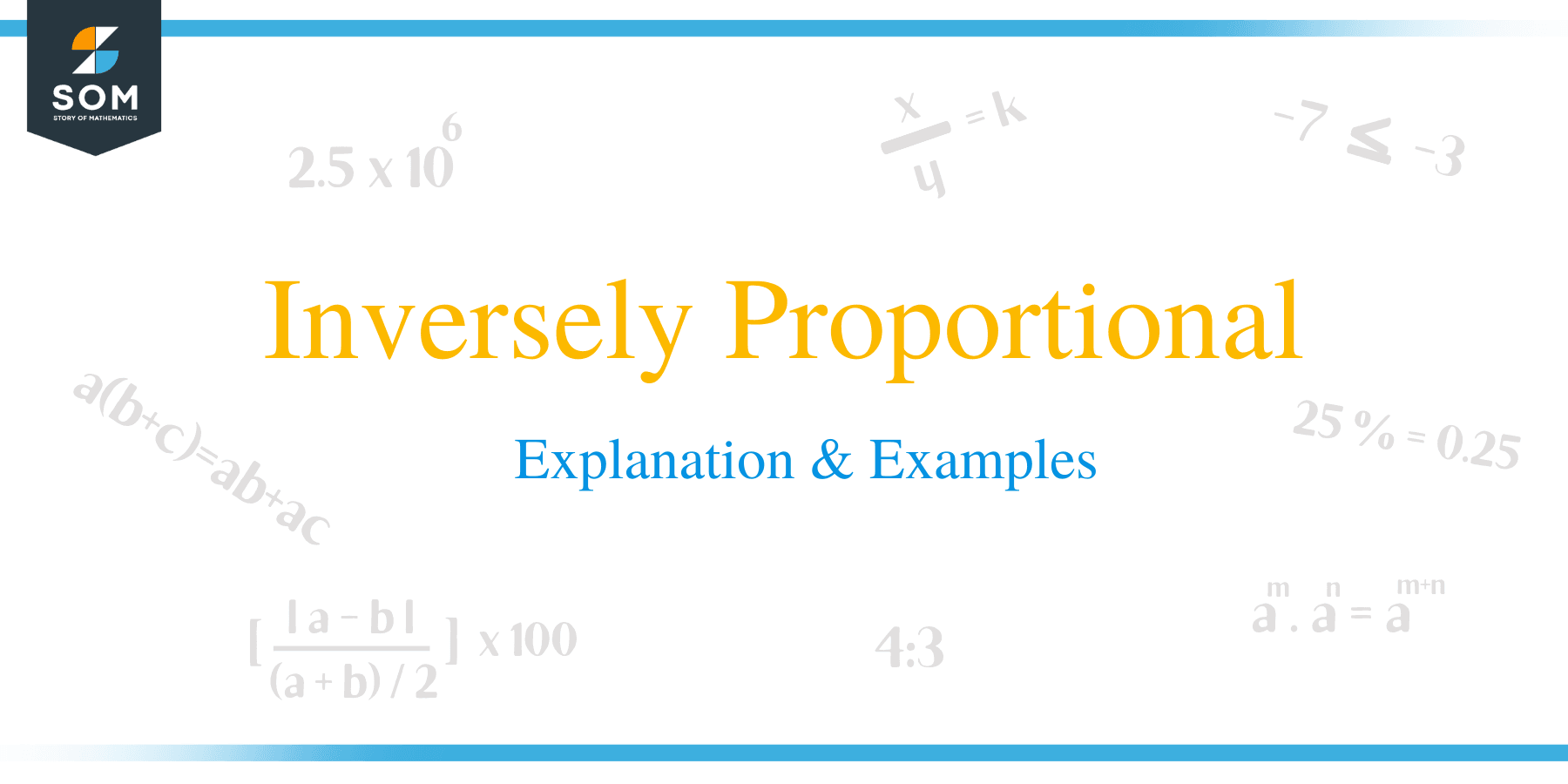# Inversely Proportional – Explanation & Examples

##What does Inversely Proportional Mean?

In our day-to-day life, we frequently encounter situations where the variation in values of a certain quantity is influenced by the variation in values of another quantity.

For example, the siren of an approaching fire engine or ambulance is becoming as louder as the vehicle nears you and as quieter as it gets farther away. You noticed that the less the distance between you and the vehicle, the louder the siren and the more the distance, the quieter the siren becomes. This type of situation is referred to as inverse proportion or sometimes indirect proportion.

Direct and indirect proportion are two concepts that we are all familiar with, just maybe not on a mathematical level. Direct and inverse proportion are both used to show how two quantities are related to each other.

In this article, we are going to learn about inverse and indirect proportion and how these concepts are important to real life situations. but before we begin, let’s remind ourselves about the concept of direct proportion.

### Direct proportion

Two variables a and b are said to be directly proportional if an increase in one variable cause the other variable to increases too and vice versa. This mean that in direct proportion, the ratio of the corresponding values of variables remains constant. In this case if the values of b; b1, b2 corresponds to the values of a; a1, a2 respectively then, their ratio is constant;

a1//b1 = a2 /b2

Direct proportion is represented the proportional sign ‘∝’ as a ∝ b.  The formula for direct variation is given by:

a/ b = k

where k is called the constant of proportionality.

### Inverse proportion

In contrast with direct proportion, where one quantity varies directly as per changes in other quantity, in inverse proportion, an increase in one variable causes a decrease in the other variable, and vice versa. Two variables a and b are said to be inversely proportional if; a∝1/b. In this case, an increase in variable b causes a reduction in the value of variable a. Similarly, a decrease in variable b causes an increment in the value of variable a.

## Indirectly Proportional Formula

If variable a is inversely proportional to variable b then, this can be represented in the formula:

a∝1/b

ab = k; where k is the proportional constant.

To set up an inverse proportional equation, the following steps are considered:

• Write down the proportional relationship
• Write the equation using the proportional constant
• Now find the value of the constant using the given values
• Substitute the value of the constant in the equation.### Real life examples of the concept of inverse proportion

• The time taken by a certain number of workers to accomplish a piece of work inversely varies as the number of workers at work. This means that, the lesser the number of workers, the more time taken to finish the work and vice versa.
• The speed of a moving vessel such as a train, vehicle or ship inversely varies as the time taken to cover a certain distance. The higher the speed, the lesser the time taken to cover the distance.

Example 1

It takes 8 days for 35 laborers to harvest coffee on a plantation. How long will 20 laborers take to harvest coffee on the same plantation.

Solution

• 35 laborers harvest coffee in 8 days

Duration taken by one worker = (35 × 8) days

• Now calculate the duration taken by 20 workers

= (35 × 8)/20

= 14 days
Therefore, 20 laborers will take 14 days.

Example 2

It takes 28 days for 6 goats or 8 sheep to graze a field. How long will 9 goats and 2 sheep take to graze the same field.
Solution
6 goats = 8 sheep
⇒ 1 goat = 8/6 sheep
⇒ 9 goats ≡ (8/6 × 9) sheep = 12 sheep
⇒ (9 goats + 2 sheep) ≡ (12 sheep + 2 sheep) = 14 sheep

Now, 8 sheep => 28 days

One sheep will graze in (28 × 8) days

⇒ 14 sheep will take (28 × 8)/14 days
= 16 days
Hence, 9 goats and 2 sheep will take 16 days to graze the field.

Example 3

Nine taps can fill a tank in four hours. How long will it take twelve taps of similar flowrate to fill the same tank?

Solution

Let the ratios;

x1/x2 = y2/ y1

⇒ 9/x = 12/4

x = 3

Therefore, 12 taps will take 3 hours to fill the tank.

### Practice Questions

1. An army barrack has enough food to feed $120$ soldiers for $80$ days. How long will the food last when $30$ more soldiers are added to the camp after $20$ days?

2. Twelve faucets share an equal flow rate and each faucet can fill a tank in $40$ minutes. If two faucets are not working, how long will it take for the remaining faucets to fill the same tank?

3. The total weekly salary for $12$ laborers working for $16$ hours a day is $\$ 16800$. What will be the weekly salary of$18$laborers working for$12$hours a day? 4. John’s company supplies milk for the nearby school’s cafeteria. He noticed that$80$students consume$1200$liters of milk in$30$days. He’s now trying to predict the number of students that can consume$1680$liters of milk in$28$days. How many students should he account for? 5. In a startup company, either$8$senior developers or$12$junior developers can finish a project in$96$days. How long will it take for$6$senior developers and$8\$ junior developers to complete the same project?